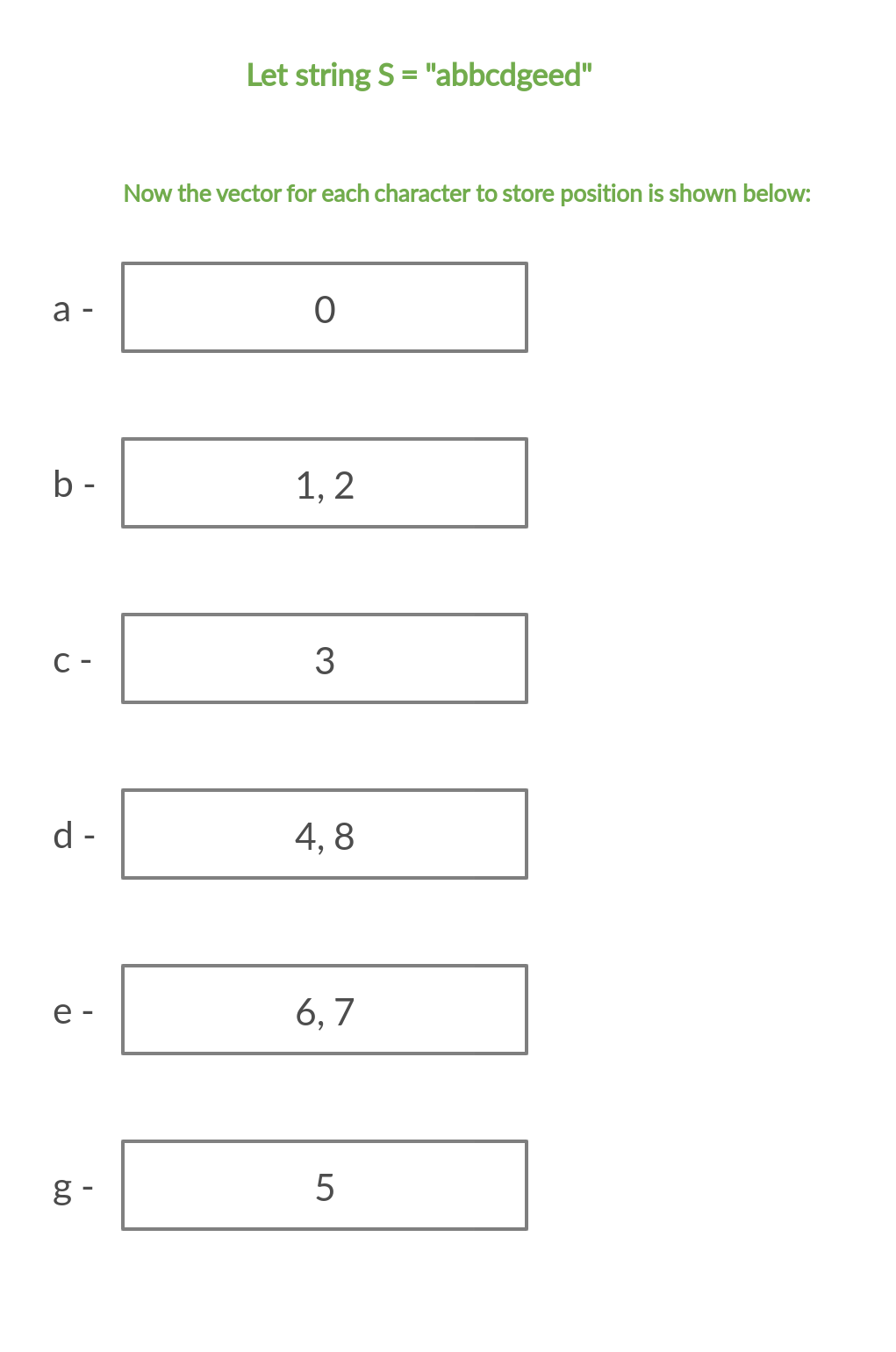Related Articles

# Count of distinct characters in a substring by given range for Q queries

• Difficulty Level : Medium
• Last Updated : 03 Jun, 2021

Given a string S consisting of lower case alphabets of size N and Q queries in the range [L, R], the task is to print the count of distinct characters in the substring by given range for each query.

Examples:

Input: S = “geeksforgeeks”, Q[][] = {{0, 4}, {3, 7}}
Output:

Explanation:
Distinct characters in substring S[0:4] are ‘g’, ‘e’, ‘k’ and ‘s’
Distinct characters in substring in S[3:7] are ‘k’, ‘s’ ‘f’, ‘o’ and ‘r’

Input: S = “geeksforgeeksisacomputerscienceportal”, Q[][] = {{0, 10}, {15, 18}, {12, 20}}
Output:

Naive Approach: The idea is to use hashing to maintain the frequency of each character in the given substring and then count the characters with a frequency equal to 1.
Below is the implementation of the above approach:

## C++

 `// C++ Program for Naive Approach` `#include ``using` `namespace` `std;` `void` `findCount(string s, ``int` `L, ``int` `R)``{``    ``// counter to count distinct char``    ``int` `distinct = 0;` `    ``// Initializing frequency array to``    ``// count characters as the appear in``    ``// substring S[L:R]``    ``int` `frequency = {};` `    ``// Iterating over S[L] to S[R]``    ``for` `(``int` `i = L; i <= R; i++) {` `        ``// incrementing the count``        ``// of s[i] character in frequency array``        ``frequency[s[i] - ``'a'``]++;``    ``}` `    ``for` `(``int` `i = 0; i < 26; i++) {` `        ``// if frequency of any character is > 0``        ``// then increment the counter``        ``if` `(frequency[i] > 0)``            ``distinct++;``    ``}` `    ``cout << distinct << endl;``}` `/* Driver code*/``int` `main()``{``    ``string s = ``"geeksforgeeksisacomputerscienceportal"``;``    ``int` `queries = 3;``    ``int` `Q[queries] = { { 0, 10 },``                          ``{ 15, 18 },``                          ``{ 12, 20 } };` `    ``for` `(``int` `i = 0; i < queries; i++)``        ``findCount(s, Q[i], Q[i]);` `    ``return` `0;``}`

## Java

 `// Java program for the naive approach``class` `GFG{` `static` `void` `findCount(String s, ``int` `L,``                                ``int` `R)``{``    ` `    ``// Counter to count distinct char``    ``int` `distinct = ``0``;` `    ``// Initializing frequency array``    ``// to count characters as the``    ``// appear in subString S[L:R]``    ``int` `[]frequency = ``new` `int``[``26``];` `    ``// Iterating over S[L] to S[R]``    ``for``(``int` `i = L; i <= R; i++)``    ``{``       ` `       ``// Incrementing the count of s[i]``       ``// character in frequency array``       ``frequency[s.charAt(i) - ``'a'``]++;``    ``}` `    ``for``(``int` `i = ``0``; i < ``26``; i++)``    ``{``       ` `       ``// If frequency of any character``       ``// is > 0 then increment the counter``       ``if` `(frequency[i] > ``0``)``           ``distinct++;``    ``}``    ``System.out.print(distinct + ``"\n"``);``}` `// Driver code``public` `static` `void` `main(String[] args)``{``    ``String s = ``"geeksforgeeksisa"` `+``               ``"computerscienceportal"``;``    ``int` `queries = ``3``;``    ``int` `Q[][] = { { ``0``, ``10` `},``                  ``{ ``15``, ``18` `},``                  ``{ ``12``, ``20` `} };` `    ``for``(``int` `i = ``0``; i < queries; i++)``       ``findCount(s, Q[i][``0``], Q[i][``1``]);``}``}` `// This code is contributed by sapnasingh4991`

## Python3

 `# Python3 program for Naive Approach` `def` `findCount(s, L, R):``    ` `    ``# Counter to count distinct char``    ``distinct ``=` `0` `    ``# Initializing frequency array to``    ``# count characters as the appear in``    ``# substring S[L:R]``    ``frequency ``=` `[``0` `for` `i ``in` `range``(``26``)]` `    ``# Iterating over S[L] to S[R]``    ``for` `i ``in` `range``(L, R ``+` `1``, ``1``):``        ` `        ``# Incrementing the count of s[i]``        ``# character in frequency array``        ``frequency[``ord``(s[i]) ``-` `ord``(``'a'``)] ``+``=` `1` `    ``for` `i ``in` `range``(``26``):``        ` `        ``# If frequency of any character is > 0``        ``# then increment the counter``        ``if` `(frequency[i] > ``0``):``            ``distinct ``+``=` `1` `    ``print``(distinct)` `# Driver code``if` `__name__ ``=``=` `'__main__'``:``    ` `    ``s ``=` `"geeksforgeeksisacomputerscienceportal"``    ``queries ``=` `3``    ``Q ``=` `[ [ ``0``, ``10` `],``          ``[ ``15``, ``18` `],``          ``[ ``12``, ``20` `] ]` `    ``for` `i ``in` `range``(queries):``        ``findCount(s, Q[i][``0``], Q[i][``1``])` `# This code is contributed by Bhupendra_Singh`

## C#

 `// C# program for the naive approach``using` `System;``class` `GFG{` `static` `void` `findCount(String s, ``int` `L,``                                ``int` `R)``{``    ` `    ``// Counter to count distinct char``    ``int` `distinct = 0;` `    ``// Initializing frequency array``    ``// to count characters as the``    ``// appear in subString S[L:R]``    ``int` `[]frequency = ``new` `int``;` `    ``// Iterating over S[L] to S[R]``    ``for``(``int` `i = L; i <= R; i++)``    ``{``        ` `        ``// Incrementing the count of s[i]``        ``// character in frequency array``        ``frequency[s[i] - ``'a'``]++;``    ``}` `    ``for``(``int` `i = 0; i < 26; i++)``    ``{``            ` `        ``// If frequency of any character``        ``// is > 0 then increment the counter``        ``if` `(frequency[i] > 0)``            ``distinct++;``    ``}``    ``Console.Write(distinct + ``"\n"``);``}` `// Driver code``public` `static` `void` `Main(String[] args)``{``    ``String s = ``"geeksforgeeksisa"` `+``               ``"computerscienceportal"``;``    ``int` `queries = 3;``    ``int` `[,]Q = {{ 0, 10 },``                ``{ 15, 18 },``                ``{ 12, 20 }};` `    ``for``(``int` `i = 0; i < queries; i++)``    ``findCount(s, Q[i, 0], Q[i, 1]);``}``}` `// This code is contributed by sapnasingh4991`

## Javascript

 ``
Output:
```7
4
8```

Time Complexity: O(Q×N)

Efficient Approach: An efficient approach would be to store the position of each character as they appear in the string in an array. For each given query we will iterate over all the 26 lower case alphabets. If the current letter is in the substring S[L: R], then the first element greater than or equal L in the corresponding position vector should exist and be less than or equal to R i.e, there must be a position value between L and R denoting the presence of the alphabet in the query range.
For example:Below is the implementation of the above approach:

## C++

 `// C++ Program for Efficient Approach``// to count the no. of distinct characters``// in a substring using STL` `#include ``using` `namespace` `std;` `// vector of vector to store``// position of all characters``// as they appear in string``vector > v(26);` `void` `build_position_vector(string s)``{``    ``for` `(``int` `i = 0; i < s.size(); i++) {` `        ``// inserting position of``        ``// character as they appear``        ``v[s[i] - ``'a'``].push_back(i);``    ``}``}` `void` `findCount(string s, ``int` `L, ``int` `R)``{` `    ``build_position_vector(s);` `    ``// counter to count distinct char``    ``int` `distinct = 0;` `    ``// iterating over all 26 characters``    ``for` `(``int` `i = 0; i < 26; i++) {` `        ``// Finding the first element``        ``// which is greater than or equal to L``        ``auto` `first = lower_bound(``            ``v[i].begin(),``            ``v[i].end(), L);` `        ``// Check if first pointer exists``        ``// and is less than or equal to R``        ``if` `(first != v[i].end()``            ``&& *first <= R) {``            ``distinct++;``        ``}``    ``}` `    ``cout << distinct << endl;``}` `/* Driver code*/``int` `main()``{``    ``string s = ``"geeksforgeeksisacomputerscienceportal"``;``    ``int` `queries = 3;``    ``int` `Q[queries] = { { 0, 10 },``                          ``{ 15, 18 },``                          ``{ 12, 20 } };` `    ``for` `(``int` `i = 0; i < queries; i++)``        ``findCount(s, Q[i], Q[i]);` `    ``return` `0;``}`
Output:
```7
4
8```

Time Complexity: O(N + Q logN)

My Personal Notes arrow_drop_up